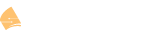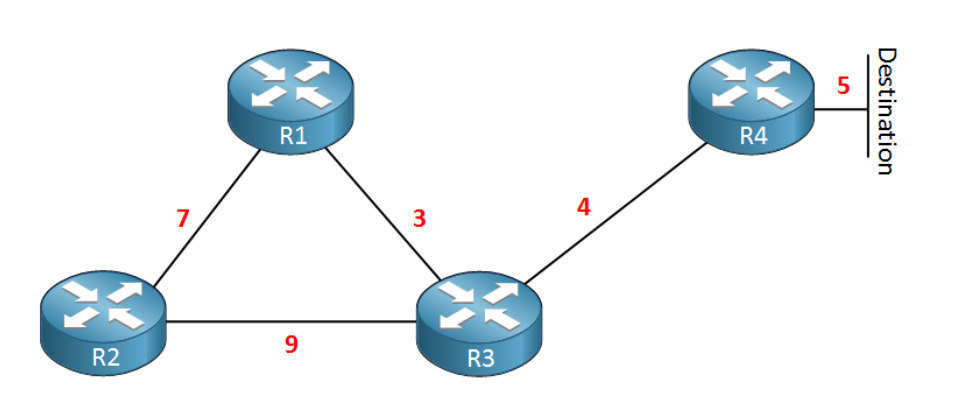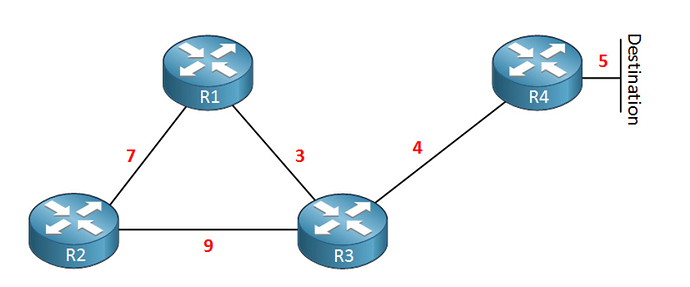# Introduction to EIGRP

Hello Hany

Can you let us know which topology you labbed up so that we can help you out? Rene has three different tolologies in this lesson.

Thanks!

Laz

Hello again Hany

So, in the original setup, with split horizon functioning, R3 will advertise the destination network to R1 and R2, however, R1 and R2 will not re-advertise the destination back to R3 because of the split horizon rule. This is why you can’t see these routes in the topology table. Now I noticed that @ReneMolenaar had the following list of advertisements that would occur:

R4 will advertise the destination network to R3.
R3 will advertise the network to R1 and R2.
R1 will advertise the network to R2.
R2 will advertise the network to R1.
R1 will advertise this network back to R3.
R2 will advertise this network back to R3.

The last two should not occur, since the split horizon rule is in effect. I will let @ReneMolenaar know about this issue.

If you forced R3 not to advertise to R1, then R1 will learn of the destination from R2 and R2 from R3. So, R2 can advertise to R3 without violating the split horizon rule.

Under normal circumstances, you shouldn’t need to manually change what R3 is advertising to R1, split horizon will take care of avoiding loops.

I hope this has been helpful!

Laz

Hello Laz,

I think you mean R1 can advertise to R3 without violating split horizon rule. There is no way R2 will advertise the network to R3 as it has originally learned the route through it and since split horizon is on

Hello Hany

Yes you are correct, the phrase should read “So, R1 can advertise to R3 without violating the split horizon rule”

Thanks!

Laz

Hello,

I still have doubt to get Advertised distance and Feasible distance as per topology. As mentioned that Advertised distance: How far the destination is away for your neighbor and Feasible distance: The total distance to the destination.

I followed the given steps:

R4 will advertise the destination network to R3.
R3 will advertise the network to R1 and R2.
R1 will advertise the network to R2.
R2 will advertise the network to R1.
R1 will advertise this network back to R3.
R2 will advertise this network back to R3.

But i am not getting same value as mentioned in result.

As per my understanding the result is mentioned below:

1. R4 will advertise the destination Network to R3 which is 5 so AD for R3= 5
2. Now R3 will advertise to R1 and R2 which is 9 so as of now AD for R1 and R2 will be 9 (5+4)
3. R1 will advertise to R2 what it has learned from R3 so AD for R2= 12 (5+4+3)
4. R2 will advertise to R1 what it has learned from R3 so AD fro R1=18 (5+4+9)
5. R1 will advertise back to R3 what it has learned from R2 so AD for R3=25 (5+4+9+7)
6. R2 will advertise back to R3 what it has learned from R1 so AD for R3=19 (5+4+3+7)

We have two AD values for each router here so now which Advertised distance (AD) would be selected.

And how R4 Feasible distance would be 9 ? as it is directly connected to destination.

R3 must have AD=5 and FD=9 but in case R4 i am confused !

1 Like

Hello Abhishek

I understand your confusion, and it is complicated further because of the names that are used (advertised and feasible distance etc.) I will attempt to clear it up.

In this example, we are looking at the network from the point of view of R3. So imagine yourself physically at that location and attempt to view the network from that perspective.

A few definitions:

**Advertised Distance**: the distance that OTHER routers are at from the destination. The OTHER routers advertise this information to R3 and R3 populates its topology accordingly.
**Feasible Distance**: the distance to the destination via each possible route as recorded in the topology of R3.

Remember, we are looking at the network from R3’s perspective.

1. Advertised distance from R4 to the destination is 5. Why? Because R4 informs R3 that to reach the destination, it has a distance of 5. Straightforward.
3. Advertised distance from R2 to the destination is 19. Again, like before, R2 has two routes to the destination: R2–>R3–>R4–>Destination and R2–>R1–>R3–>R4–>Destination with distances at 9+4+5=18 and 1+3+4+5=19. Again, because of split horizon, the first is not used, so the advertised distance is 19.

For Feasible Distances from R3:

1. For the route via R4, the Feasible distance is 4+5=9
2. For the route via R1, the Feasible distance is 3+7+9+4+5=28
3. For the route via R2 the Feasible distance is 9+7+3+4+5 = 28

Yes, for R1 and R2 we have two Advertised Distances. But the only ones that will reach R3 are those that have been learned from a different interface. Remember split horizon. So only one is chosen.

Remember we are looking at the network from R3s perspective. So the Feasible Distance of 9 indicates that the route to the destination FROM R3 via R4 will have a total distance of 4+5=9.

I hope this has been helpful!

Laz

Hello Laz,

Thanks for clarification. Now i am getting your point.

Please make me correct if i am wrong. Now i am taking AD and FD value from R2’s perspective.

1. Advertised distance from R3 is 9 (Path R3->R4) directly
2. Advertise distance from R1 is 25 (Path R1->R2->R3->R4->Destnation). Second path would not be selected as per split horizon rule which is (R1->R3->R4->Destination).

Feasible distance

1. FD from R3 is = 9+4+5=18

2. FD from R1 is = 25+7= 32

Thanks,
Abhishek Saini

Hello again Abhishek

You’ve almost got it. I think you understand it, but you just misread some costs.

From R2’s perspective:

1. Advertised distance of R3 to destination is 4+5=9 (Path R3–>R4–>Dest). You are correct here.
2. Advertised distance of R1 to destination is 3+4+5=12 (Path R1–>R3–>R4–> Dest). The second path that will not be included would have been R1–>R2–>R3–>R4–Dest. which has a distance of 7+9+4+5=25. This will not be used as per split horizon rule. Remember, we are looking at the network from the point of view of R2 now.

Feasible Distance

1. FD of R2 VIA R3 is 9+4+5=18 You are correct here.
2. FD of R2 VIA R1 is 7+3+4+5=19

I hope this has been helpful!

Laz

Hello Laz,

I believe, Now i got the point.

Thank you so much for your help.

Just for my practice i found out AD and FD values from R1 perspective.

Advertised distance from R3 is 9 (Path R3->R4) directly
Advertise distance from R1 is 18 (Path R2->R3->R4->Destnation). Second path would not be selected as per split horizon rule which is (R2->R1->R3->R4->Destination).

Feasible distance

FD from R3 is = 9+3=12
FD from R2 is = 18+7=25

Thanks,
Abhishek

Hello Abhishek

Yes you’ve got it! I assume however that you R2 and not R1 in the following statement:

Good work!

Laz

Hi Rene
In this topologyI don’t understand where all of this value come from it see so complicateDo u have better way help me to understand this ?

Hi Heng,

These values are metric values that I made up. On real routers running EIGRP, you will see very large numbers for the metrics which makes it harder to explain.

The advertised distance is the metric that a router has received from its neighbor. The feasible distance is the total distance to get to the destination. In other words, the advertised distance + the metric on your own interface.

To understand the values of the table in your screenshot, it’s best to fill in the topology tables of R1, R2, and R3 yourself first:

R3’s topology table:

R1
R2
R4

R1’s topology table:

R2
R3

R2’s topology table:

R1
R3

For example, here’s the first entry for R3:

R1
R3
R4 5 9

In the topology table of R3, you see an entry for the advertised distance of 5. That’s what R4 advertises to R3. R3 adds its own metric on the interface that connects to R4 (4) to the advertised distance. 4+5 = 9 so that becomes the feasible distance.

R3 advertises this entry to R1 and R2.

R1’s topology table looks like this:

R2
R3 9 12

R3 advertises a metric of 9 so that’s what we add in the advertised distance. It adds its own interface metric (3) to this value, so the feasible distance becomes 12.

Now see if you can enter the remaining values yourself. Keep in mind that that the route is also advertised from R3 > R1 > R2 and back to R3. It’s also advertised from R3 > R2 > R1 > R3.

hi rene i have question regarding the below example in the picture:when R3 received 25AD from R1 and 19AD from R2 will R3 send those values back to R4?

R3 will share its own advertised distance to the destiation with R4 and not the advertised distance reported by R1 and R2. So R3 will share an AD of 9 to the destination with R4.

In general, in EIGRP a router will share its own AD with other routers and the the AD reported by other routers.

I hope this has been helpful!

Laz

1 Like

thank you for your reply, from my understanding, router will share the reported AD from other router which means when R3 receives (AD=25 from R1) and (AD=19 from R2) so as a result --> R3 should send both AD’s to R4 and this does not make sense!

In reality, when R3 receives an update from R1 or R2 about the destination behind R4, it won’t install them since these don’t pass the feasibility condition (AD of the feasible successor has to be lower than FD of successor).

R3 will only advertise its successor route to other neighbors. In this topology, R4 is the successor route so normally R3 would advertise this route to R4. However, because of split horizon (don’t advertise a route to a neighbor if you learned that route from the neighbor)…this route is not advertised.

It might be helpful to see all of this in action. Connect four routers like in the topology picture, then load these four configs:

R1-show-run-2018-01-17-15-58-39-clean.txt (270 Bytes)
R2-show-run-2018-01-17-15-58-43-clean.txt (270 Bytes)
R3-show-run-2018-01-17-15-58-46-clean.txt (366 Bytes)
R4-show-run-2018-01-17-15-58-50-clean.txt (261 Bytes)

If you want to see the EIGRP updates, you can start Wireshark and use this filter:

`eigrp.opcode == 1`

It will show you the route that is advertised and the metric.

Seeing this in action probably makes it easier to understand.

Rene

1 Like

Thank you for the clarification, i will try to demonstrate this test in lab , when i see the eigrp exchange packets in live i will get the full picture.

Hi Rene, The topic is very interesting, Thank you.

Hello Rene

can you tell me link where i find below topic

LISP encapsulation principles supporting EIGRP OTP